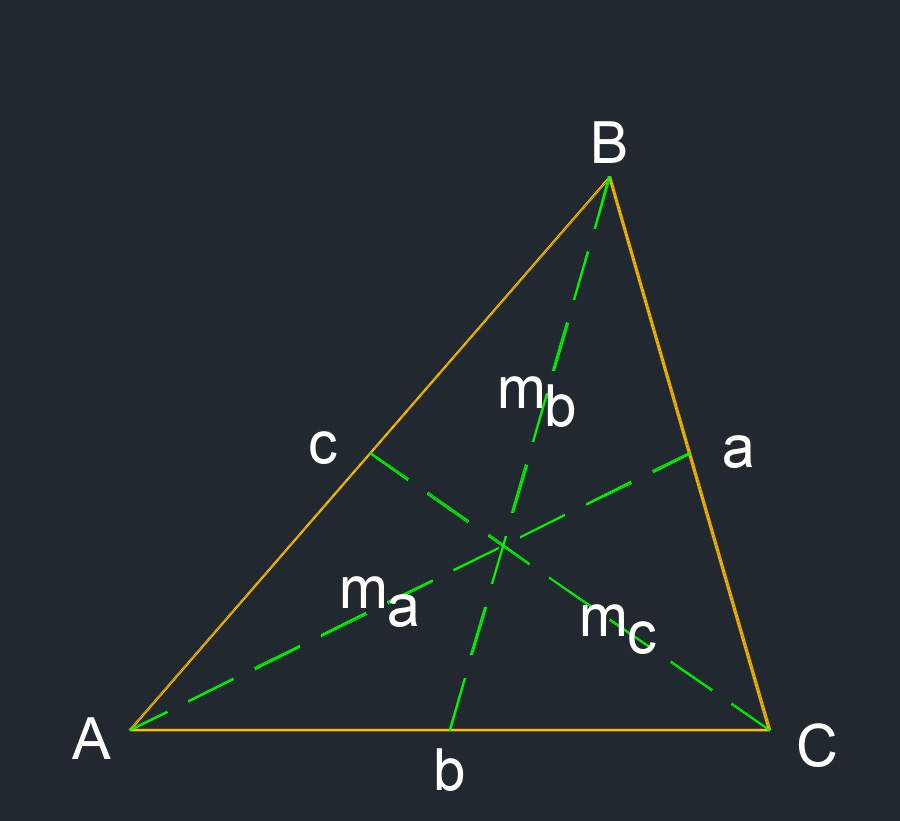# Scalene Triangle

on . Posted in Plane Geometry

•Scalene triangle (a two-dimensional figure) is where all three sides are different lengths and all three angles are different angles.
• Angle bisector of a scalene triangle is a line that splits an angle into two equal angles.
• Median of a scalene triangle is a line segment from a vertex (coiner point) to the midpoint of the opposite side.
• Circumcircle is a circle that passes through all the vertices of a two-dimensional figure.
• Inscribed circle is the Iargest circle possible that can fit on the inside of a two-dimensional figure.
• Semiperimeter is one half of the perimeter.
• x + y + z = 180°
• Height:  $$h_a$$,  $$h_b$$,  $$h_c$$
• Median:  $$m_a$$,  $$m_b$$,  $$m_c$$  -  A line segment from a vertex (corner point) to the midpoint of the opposite side
• Angle bisectors:  $$t_a$$,  $$t_b$$,  $$t_c$$  -  A line that splits an angle into two equal angles
• 3 edges
• 3 vertexs## Angle bisector of a Scalene Triangle formulas

$$\large{ t_a = 2\;b \;c \; cos \; \frac { \frac {A}{2} } { b \;+\; c } }$$

$$\large{ t_a = \sqrt { b\;c \; \frac { 1 \;- \; a^2 }{ \left( b \;+\; c \right)^2 } } }$$

Symbol English Metric
$$\large{ t_a }$$ = angle bisector $$\large{ in}$$ $$\large{ mm }$$
$$\large{ \theta }$$ = angle $$\large{deg}$$ $$\large{rad}$$
$$\large{ a, b, c }$$ = edge $$\large{ in}$$ $$\large{ mm }$$## Area of a Scalene Triangle formulas

$$\large{ A_{area} = \frac {h\;b} {2} }$$

$$\large{ A_{area} = a\;b\; \frac {\sin y} {2} }$$

Symbol English Metric
$$\large{ A_{area} }$$ = area $$\large{ in^2}$$ $$\large{ mm^2}$$
$$\large{ a, b, c }$$ = edge $$\large{ in}$$ $$\large{ mm }$$

## Circumcircle of a Scalene Triangle formulas

$$\large{ R = \sqrt { \frac { a^2 \; b^2 \; c^2 } { \left( a \;+\; b \;+\; c \right) \; \left( - a + b + c \right) \; \left( a \;-\; b \;+\; c \right) \; \left( a \;+\; b \;-\; c \right) } } }$$

$$\large{ R = \frac { a \; b \; c } { 4 \; \sqrt { s\; \left( s \;-\; a \right) \; \left( s \;-\; b \right) \; \left( s \;-\; c \right) } } }$$

Symbol English Metric
$$\large{ R }$$ = outcircle $$\large{ in}$$ $$\large{ mm }$$
$$\large{ a, b, c }$$ = edge $$\large{ in}$$ $$\large{ mm }$$
$$\large{ s }$$ = semiperimeter $$\large{ in}$$ $$\large{ mm }$$## Height of a Scalene Triangle formulas

$$\large{ h_a = c \; sin\; B }$$

$$\large{ h_a = b \; sin\; C }$$

$$\large{ h_a = 2\; \frac {A_{area}}{a} }$$

Symbol English Metric
$$\large{ h_a }$$ = height $$\large{ in}$$ $$\large{ mm}$$
$$\large{ B, C }$$ = angle $$\large{ deg}$$ $$\large{ rad}$$
$$\large{ A_{area} }$$ = area $$\large{ in^2}$$ $$\large{ mm^2 }$$
$$\large{ a, b, c }$$ = edge $$\large{ in}$$ $$\large{ mm }$$

## Inscribed Circle of a Scalene Triangle formula

$$\large{ r = \sqrt { \frac { \left( s \;-\; a \right) \; \left( s \;-\; b \right) \; \left( s \;-\; c \right) } { s } } }$$
Symbol English Metric
$$\large{ r }$$ = incircle $$\large{ in}$$ $$\large{ mm }$$
$$\large{ a, b, c }$$ = edge $$\large{ in}$$ $$\large{ mm }$$## Median of a Scalene Triangle formula

$$\large{ m_a = \sqrt { \frac { 2\;b^2 \;+\; 2\;c^2 \;-\; a^2 } {2} } }$$
Symbol English Metric
$$\large{ m_a }$$ = median $$\large{ in}$$ $$\large{ mm }$$
$$\large{ a, b, c }$$ = edge $$\large{ in}$$ $$\large{ mm }$$## Perimeter of a Scalene Triangle formula

$$\large{ P = a + b + c }$$
Symbol English Metric
$$\large{ P }$$ = perimeter $$\large{ in}$$ $$\large{ mm }$$
$$\large{ a, b, c }$$ = edge $$\large{ in}$$ $$\large{ mm }$$## Semiperimeter of a Scalene Triangle formula

$$\large{ s = \frac{ a \;+\; b \;+\; c }{ 2 } }$$
Symbol English Metric
$$\large{ s }$$ = semiperimeter $$\large{ in}$$ $$\large{ mm }$$
$$\large{ a, b, c }$$ = edge $$\large{ in}$$ $$\large{ mm }$$## Side of a Scalene Triangle formulas

$$\large{ a = P - b - c }$$

$$\large{ a = 2\; \frac {A_{area}} {b\;\sin y} }$$

$$\large{ b = P - a - c }$$

$$\large{ b = 2\; \frac {A_{area}}{h} }$$

$$\large{ c = P - a - b }$$

Symbol English Metric
$$\large{ a, b, c }$$ = edge $$\large{ in}$$ $$\large{ mm }$$
$$\large{ A_{area} }$$ = area $$\large{ in^2}$$ $$\large{ mm^2 }$$
$$\large{ P }$$ = perimeter $$\large{ in}$$ $$\large{ mm }$$Tags: Triangle Equations